ConicBundle
ConicBundle::GramSparsePSCPrimal Class Reference

represents an PSCPrimal as the sum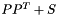of a Gram matrix and a sparse symmetric matrix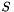More...

`#include <PSCPrimal.hxx>`

Inheritance diagram for ConicBundle::GramSparsePSCPrimal: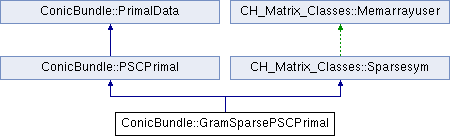## Public Member Functions

GramSparsePSCPrimal (const CH_Matrix_Classes::Sparsesym &sps, double factor=1.)
initialize to the given sparse symmetric matrix, the gram part is zero

GramSparsePSCPrimal (const GramSparsePSCPrimal &pr, double factor=1.)
copy constructor

GramSparsePSCPrimaloperator= (const CH_Matrix_Classes::Sparsesym &sdp)
assign the sparse symmetric matrix to this and set the gram part to zero

GramSparsePSCPrimaloperator= (const GramSparsePSCPrimal &sdp)
copy the information

const CH_Matrix_Classes::Matrixget_grammatrix () const
returns the matrix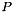giving rise to the Gram matrix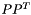PrimalDataclone_primal_data () const
returns a newly generated identical Object

int assign_Gram_matrix (const CH_Matrix_Classes::Matrix &P)
Set the grammatrix part toand set all values on the sparse support to zero (but keep this support even if it is zero now!)

int aggregate_primal_data (const PrimalData &it, double factor=1.)
if it is a GramSparsePSCPrimal or SparseSDPRimal, add factor*it to this on only the support of this sparsematrix More...

int aggregate_Gram_matrix (const CH_Matrix_Classes::Matrix &P, double factor=1.)
add factor*P*P^T to this, collecting all available information only in the sparse part, even the own Gram part. More...

virtual int scale_primal_data (double factor)
multiply/scale *this with a nonnegative factor

virtual int primal_ip (CH_Matrix_Classes::Real &value, const SparseCoeffmatMatrix &A, CH_Matrix_Classes::Integer column) const
if compatible evaluate value=ip(*this,A.column[i])Public Member Functions inherited from CH_Matrix_Classes::Sparsesym
Sparsesym ()
empty matrix

Sparsesym (const Sparsesym &A, Real d=1.)
copy constructor, *this=d*A

Sparsesym (Integer nr)
initialize to zero-matrix of size nr*nr

Sparsesym (Integer nr, Integer nz, const Integer *ini, const Integer *inj, const Real *va)
initialize to size nr*nr and nz nonzeros so that this(ini[i],inj[i])=val[i] for i=0,..,nz-1; specify only one of (i,j) and (j,i), multiple elements are summed up.

Sparsesym (Integer nr, Integer nz, const Indexmatrix &ini, const Indexmatrix &inj, const Matrix &va)
initialize to size nr*nr and nz nonzeros so that this(ini(i),inj(i))=val[i] for i=0,..,nz-1; specify only one of (i,j) and (j,i), multiple elements are summed up.

void set_init (bool)
after external initialization, call matrix.set_init(true) (not needed if CONICBUNDLE_DEBUG is undefined)

bool get_init () const
returns true if the matrix has been declared initialized (not needed if CONICBUNDLE_DEBUG is undefined)

Sparsesyminit (const Sparsesym &, Real d=1.)
initialize to *this=A*d

Sparsesyminit (const Matrix &, Real d=1.)
initialize to this=d(A+transpose(A))/2., abs(values)<tol are removed from the support

Sparsesyminit (const Indexmatrix &, Real d=1.)
initialize to this=d(A+transpose(A))/2., zeros are removed from the support

Sparsesyminit (const Symmatrix &, Real d=1.)
initialize to *this=A*d, abs(values)<tol are removed from the support

Sparsesyminit (const Sparsemat &, Real d=1.)
initialize to this=d(A+transpose(A))/2.

Sparsesyminit (Integer nr)
initialize to zero-matrix of size nr*nr

Sparsesyminit (Integer nr, Integer nz, const Integer *ini, const Integer *inj, const Real *va)
initialize to size nr*nr and nz nonzeros so that this(ini[i],inj[i])=val[i] for i=0,..,nz-1; specify only one of (i,j) and (j,i), multiple elements are summed up.

Sparsesyminit (Integer nr, Integer nz, const Indexmatrix &ini, const Indexmatrix &inj, const Matrix &va)
initialize to size nr*nr and nz nonzeros so that this(ini(i),inj(i))=val[i] for i=0,..,nz-1; specify only one of (i,j) and (j,i), multiple elements are summed up.

Sparsesyminit_support (const Sparsesym &A, Real d=0.)

void set_tol (Real t)
set tolerance for recognizing zero values to t

Sparsesym (const Matrix &, Real d=1.)
initialize to this=d(A+transpose(A))/2., abs(values)<tol are removed from the support

Sparsesym (const Indexmatrix &, Real d=1.)
initialize to this=d(A+transpose(A))/2., zeros are removed from the support

Sparsesym (const Symmatrix &, Real d=1.)
initialize to *this=A*d, abs(values)<tol are removed from the support

Sparsesym (const Sparsemat &, Real d=1.)
initialize to this=d(A+transpose(A))/2.

void dim (Integer &r, Integer &c) const
returns the number of rows in _nr and the number of columns in _nc

Integer dim () const
returns the dimension rows * columns when the matrix is regarded as a vector

Integer rowdim () const
returns the row dimension

Integer coldim () const
returns the column dimension

Integer nonzeros () const
returns the number of nonzeros in the lower triangle (including diagonal)

Mtype get_mtype () const
returns the type of the matrix, MTmatrix

Real operator() (Integer i, Integer j) const
returns value of element (i,j) of the matrix (rowindex i, columnindex j)

Real operator() (Integer i) const
returns value of element (i) of the matrix if regarded as vector of stacked columns [element (irowdim, i/rowdim)]

Real operator[] (Integer i) const
returns value of element (i) of the matrix if regarded as vector of stacked columns [element (irowdim, i/rowdim)]

const Indexmatrixget_colinfo () const
returns information on nozero diagonal/columns, k by 4, listing: index (<0 for diagonal), # nonzeros, first index in colindex/colval, index in suppport submatrix

const Indexmatrixget_colindex () const
returns the index vector of the column representation holding the row index for each element

const Matrixget_colval () const
returns the value vector of the column representation holding the value for each element

const Indexmatrixget_suppind () const
returns the index vector of the column representation holding the row index w.r.t. the principal support submatrix for each element

const Indexmatrixget_suppcol () const
returns the vector listing in ascending order the original column indices of the principal support submatrix

void get_edge_rep (Indexmatrix &I, Indexmatrix &J, Matrix &val) const
stores the nz nonzero values of the lower triangle of *this in I,J,val so that this(I(i),J(i))=val(i) for i=0,...,nz-1 and dim(I)=dim(J)=dim(val)=nz (ordered as in row representation)

int contains_support (const Sparsesym &A) const
returns 1 if A is of the same dimension and the support of A is contained in the support of *this, 0 otherwise

int check_support (Integer i, Integer j) const
returns 0 if (i,j) is not in the support, 1 otherwise

Sparsesymxeya (const Sparsesym &A, Real d=1.)
sets *this=d*A and returns *this

Sparsesymxeya (const Matrix &A, Real d=1.)
sets and returns this=d(A+transpose(A))/2. where abs(values)<tol are removed from the support

Sparsesymxeya (const Indexmatrix &A, Real d=1.)
sets and returns this=d(A+transpose(A))/2. where zeros are removed from the support

Sparsesymsupport_xbpeya (const Sparsesym &y, Real alpha=1., Real beta=0.)
returns this= alpha*y+beta(*this) restricted to the curent support of *this; if beta==0, then *this is initialized to 0 on its support first

Sparsesymoperator= (const Sparsesym &A)

Sparsesymoperator+= (const Sparsesym &v)

Sparsesymoperator-= (const Sparsesym &v)

Sparsesym operator- () const

Sparsesymoperator*= (Real d)

Sparsesymoperator/= (Real d)
ATTENTION: d is NOT checked for 0.

Sparsesymoperator= (const Matrix &A)
sets *this=(A+transpose(A))/2. removing abs(values)<tol; returns *this

Sparsesymoperator= (const Indexmatrix &A)
sets *this=(A+transpose(A))/2. removing zeros; returns *this

Sparsesymtranspose ()
transposes itself (at almost no cost)

Sparsesymxeya (const Symmatrix &A, Real d=1.)
sets and returns *this=A*d where abs(values)<tol are removed from the support

Sparsesymxeya (const Sparsemat &A, Real d=1.)
sets and returns this=d.(A+transpose(A))/2.

Sparsesymoperator= (const Symmatrix &A)
sets and returns *this=A where abs(values)<tol are removed from the support

Symmatrix operator+ (const Symmatrix &A) const

Symmatrix operator- (const Symmatrix &A) const

Sparsesymoperator= (const Sparsemat &A)
sets and returns *this=(A+transpose(A))/2.

Sparsemat sparsemult (const Matrix &A) const
compute (*this)*A and return the result in a Sparsemat

void display (std::ostream &out, int precision=0, int width=0, int screenwidth=0) const
displays a matrix in a pretty way for bounded screen widths; for variables of value zero default values are used. More...

## Protected Attributes

CH_Matrix_Classes::Matrix gramblock
the gram matrix part is gramblock*transpose(grampblock)

## Additional Inherited MembersProtected Member Functions inherited from CH_Matrix_Classes::Memarrayuser
Memarrayuser ()
if memarray is NULL, then a new Memarray is generated. In any case the number of users of the Memarray is incremented

virtual ~Memarrayuser ()
the number of users is decremented and the Memarray memory manager is destructed, if the number is zero.Static Protected Attributes inherited from CH_Matrix_Classes::Memarrayuser
static Memarraymemarray
pointer to common memory manager for all Memarrayusers, instantiated in memarray.cxx

## Detailed Description

represents an PSCPrimal as the sumof a Gram matrix and a sparse symmetric matrixGramSparsePSCPrimal is pubically derived from PSCPrimal and CH_Matrix_Classes::Sparseym, so it may be used directly like a sparse symmetric matrix, but this does then not include or involve the Gram matrix part!

## ◆ aggregate_Gram_matrix()

 int ConicBundle::GramSparsePSCPrimal::aggregate_Gram_matrix ( const CH_Matrix_Classes::Matrix & P, double factor = `1.` )
inlinevirtual

add factor*P*P^T to this, collecting all available information only in the sparse part, even the own Gram part.

This operation more or less converts this to a SparsePSCPrimal. The point is that this routine is typically only called by PSCModel when aggregating the information in a single aggregate matrix over several steps and it is pointless to try to keep any gram information in this case.

Implements ConicBundle::PSCPrimal.

## ◆ aggregate_primal_data()

 int ConicBundle::GramSparsePSCPrimal::aggregate_primal_data ( const PrimalData & it, double factor = `1.` )
inlinevirtual

if it is a GramSparsePSCPrimal or SparseSDPRimal, add factor*it to this on only the support of this sparsematrix

Even if it is a GramSparsePSCPrimal and it has a nontirival Gram matrix part, this part is only added to the sparse part of this on the support of the sparse part of this. No attempt is made to enlarge the Gram part.

Implements ConicBundle::PSCPrimal.

The documentation for this class was generated from the following file: